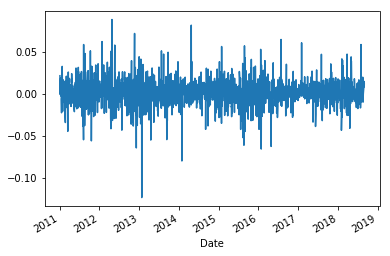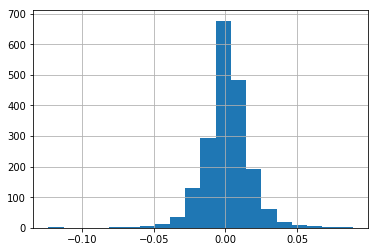# Asset pricing & factor regressions

## Asset pricing models¶

We briefly explore the mathematical and explanatory description of key asset pricing models (i.e., CAPM, Fama-French 3 Factor, Fama-French 5 factor), and how to run these models in Python.

### Capital Asset Pricing Model (CAPM)¶

All finance PhD students should have some knowledge of one of the most important topics in finance, that is the Capital Asset Pricing Model (CAPM). The CAPM model explains the relationship between systematic risk and the expected return for assets (e.g., stocks). The CAPM is used for pricing of risky assets, by generating the expected return of the asset given its riskiness, and calculating the cost of capital. Intuitively, the CAPM model tells us that the return of a risky asset is explained by the market factor (i.e., $r_m$)

$\bar{r_a} = r_f + \beta_a (\bar{r_m} - r_f)$

$\bar{r_a}$: The return of the asset. This is can be the return of the any stock (i.e., Apple, Google, Tesla) or investment portfolio (i.e., any mutual/hedge fund portfolio).
$\bar{r_f}$: The return of the risk-free asset. The risk-free asset is usually given by the US 3-month Treasury bill. It is assumed that the US government will not default on a short-term government security, thus the US 3-month Treasury bill is widely assumed in finance to be risk-free. $\bar{r_m}$: The return of the market. This is usually given by the S&P500 return as it is the largest market index in the world.

The CAPM can also be expressed mathematically in the following notation:

$r-r_f = \alpha + \beta_M (MKT-r_f)$

We will use the above notation going forward.

Note: If you are running an asset pricing model on stocks in countries outside the US, it may be more applicable to change $r_m$ ($r_f$) to the market index (short-term government security) of that country.

### Fama-French 3-factor (FF3)¶

Another very popular asset pricing model in the empirical finance literature is the Fama-French 3-factor (FF3) that was published in 1993. Nobel Laureate Eugene Fama and researcher Kenneth French found that value stocks tend to outperform growth stocks (i.e., value), and that small-cap stocks outperform large-cap stocks (i.e., size). Thus, the FF3 mode adds in size and value as risk factors to the model as shown below

$r-r_f = \alpha + \beta_M (MKT-r_f) + \beta_S SMB + \beta_v HML$

### Fama-French 5-factor (FF5)¶

In 2015, Fama-French added two more risk factors into their popular 3-factor asset pricing model to make a Fama-French 5-factor (FF5) model. This model added two 'quality' factors, namely profitability (stocks with a high operating profitability perform better) and investment (stocks of companies with high total asset growth have below average returns) factors.

$r-r_f = \alpha + \beta_M (MKT-r_f) + \beta_S SMB + \beta_v HML + \beta_r RMW + \beta_c CMA$

SMB: The return spread of small minus large stocks (size).
HML: The return of cheap minus expensive stocks (value).
RMW: The return spread of the most profitable firms minus the least profitable (profit).
CMA: The return spread of firms that invest conservatively minus aggressively (investment).

### Importing the Python modules¶

When you use the import module as short_form, this allows you to apply functions with the short_form instead. For example, when using import numpy as np, numpy.log becomes np.log.

In :
import pandas as pd
import numpy as np

import statsmodels.formula.api as sm # module for stats models
from statsmodels.iolib.summary2 import summary_col # module for presenting stats models outputs nicely


### Defining Python functions¶

We have two functions below:

1. price2ret: This converts prices to arithmetic or log returns.
2. assetPriceReg: By giving a dataframe of stock with a column named Returns, the function extracts the risk factor returns from Ken French's website and runs a CAPM, FF3, and FF5 regression
In :
def price2ret(prices,retType='simple'):
if retType == 'simple':
ret = (prices/prices.shift(1))-1
else:
ret = np.log(prices/prices.shift(1))
return ret

In :
def assetPriceReg(df_stk):
import pandas_datareader.data as web  # module for reading datasets directly from the web

df_factors.rename(columns={'Mkt-RF': 'MKT'}, inplace=True)
df_factors['MKT'] = df_factors['MKT']/100
df_factors['SMB'] = df_factors['SMB']/100
df_factors['HML'] = df_factors['HML']/100
df_factors['RMW'] = df_factors['RMW']/100
df_factors['CMA'] = df_factors['CMA']/100

df_stock_factor = pd.merge(df_stk,df_factors,left_index=True,right_index=True) # Merging the stock and factor returns dataframes together
df_stock_factor['XsRet'] = df_stock_factor['Returns'] - df_stock_factor['RF'] # Calculating excess returns

# Running CAPM, FF3, and FF5 models.
CAPM = sm.ols(formula = 'XsRet ~ MKT', data=df_stock_factor).fit(cov_type='HAC',cov_kwds={'maxlags':1})
FF3 = sm.ols( formula = 'XsRet ~ MKT + SMB + HML', data=df_stock_factor).fit(cov_type='HAC',cov_kwds={'maxlags':1})
FF5 = sm.ols( formula = 'XsRet ~ MKT + SMB + HML + RMW + CMA', data=df_stock_factor).fit(cov_type='HAC',cov_kwds={'maxlags':1})

CAPMtstat = CAPM.tvalues
FF3tstat = FF3.tvalues
FF5tstat = FF5.tvalues

CAPMcoeff = CAPM.params
FF3coeff = FF3.params
FF5coeff = FF5.params

# DataFrame with coefficients and t-stats
results_df = pd.DataFrame({'CAPMcoeff':CAPMcoeff,'CAPMtstat':CAPMtstat,
'FF3coeff':FF3coeff, 'FF3tstat':FF3tstat,
'FF5coeff':FF5coeff, 'FF5tstat':FF5tstat},
index = ['Intercept', 'MKT', 'SMB', 'HML', 'RMW', 'CMA'])

dfoutput = summary_col([CAPM,FF3, FF5],stars=True,float_format='%0.4f',
model_names=['CAPM','FF3','FF5'],
info_dict={'N':lambda x: "{0:d}".format(int(x.nobs)),
regressor_order = ['Intercept', 'MKT', 'SMB', 'HML', 'RMW', 'CMA'])

print(dfoutput)

return results_df


## Script to run asset pricing regressions on stock returns¶

Changing file directories to read CSV files

In :
from pathlib import Path
import sys
import os

home = str(Path.home())

if sys.platform == 'linux':
inputDir = '/datasets/stocks/'
elif sys.platform == 'win32':
inputDir = '\\datasets\stocks\\'

fullDir = home+inputDir


Reading in stock data, removing volume information, and plotting prices

In :
stkName = 'AAPL'
fileName = 'stk_' + stkName + '.csv'


Out:
Open High Low Close Adj Close Volume
Date
2010-12-31 46.135715 46.211430 45.901428 46.080002 40.838676 48377000
2011-01-03 46.520000 47.180000 46.405716 47.081429 41.726177 111284600
2011-01-04 47.491428 47.500000 46.878571 47.327145 41.943951 77270200
2011-01-05 47.078571 47.762856 47.071430 47.714287 42.287071 63879900
2011-01-06 47.817142 47.892857 47.557144 47.675713 42.252876 75107200
In :
df_stk.drop(['Volume'],axis=1,inplace=True)
df_stk.plot()

Out:
<matplotlib.axes._subplots.AxesSubplot at 0x7f426725d588>

Calculating simple returns from stock prices

In :
df_stk['Returns'] = price2ret(df_stk[['Adj Close']])
df_stk = df_stk.dropna()

Out:
Open High Low Close Adj Close Returns
Date
2011-01-03 46.520000 47.180000 46.405716 47.081429 41.726177 0.021732
2011-01-04 47.491428 47.500000 46.878571 47.327145 41.943951 0.005219
2011-01-05 47.078571 47.762856 47.071430 47.714287 42.287071 0.008180
2011-01-06 47.817142 47.892857 47.557144 47.675713 42.252876 -0.000809
2011-01-07 47.712856 48.049999 47.414288 48.017143 42.555470 0.007162
In :
df_stk['Returns'].plot()

Out:
<matplotlib.axes._subplots.AxesSubplot at 0x7f4266dd3d30>In :
df_stk['Returns'].hist(bins=20)

Out:
<matplotlib.axes._subplots.AxesSubplot at 0x7f4264d5a6d8>Running risk factor regressions

In :
df_regOutput = assetPriceReg(df_stk)

===========================================
CAPM      FF3        FF5
-------------------------------------------
Intercept   -0.0006*  -0.0007**  -0.0007**
(0.0003)  (0.0003)   (0.0003)
MKT         0.9027*** 0.9813***  0.9704***
(0.0338)  (0.0343)   (0.0362)
SMB                   -0.2612*** -0.1170*
(0.0607)   (0.0613)
HML                   -0.6568*** -0.0560
(0.0707)   (0.0802)
RMW                              0.6695***
(0.1216)
CMA                              -1.4146***
(0.1517)
N           1930      1930       1930
===========================================
Standard errors in parentheses.
* p<.1, ** p<.05, ***p<.01


## Converting iPython notebook to Python code¶

In :
!jupyter nbconvert --to python fin-econ_assetpricing_regression.ipynb

[NbConvertApp] Converting notebook fin-econ_assetpricing_regression.ipynb to python
[NbConvertApp] Writing 8054 bytes to fin-econ_assetpricing_regression.py

##### Acknowledgements¶

I'd like to acknowledge Lauchlan Michalski for his work on downloading the datasets and writing the basic regression framework used in this post